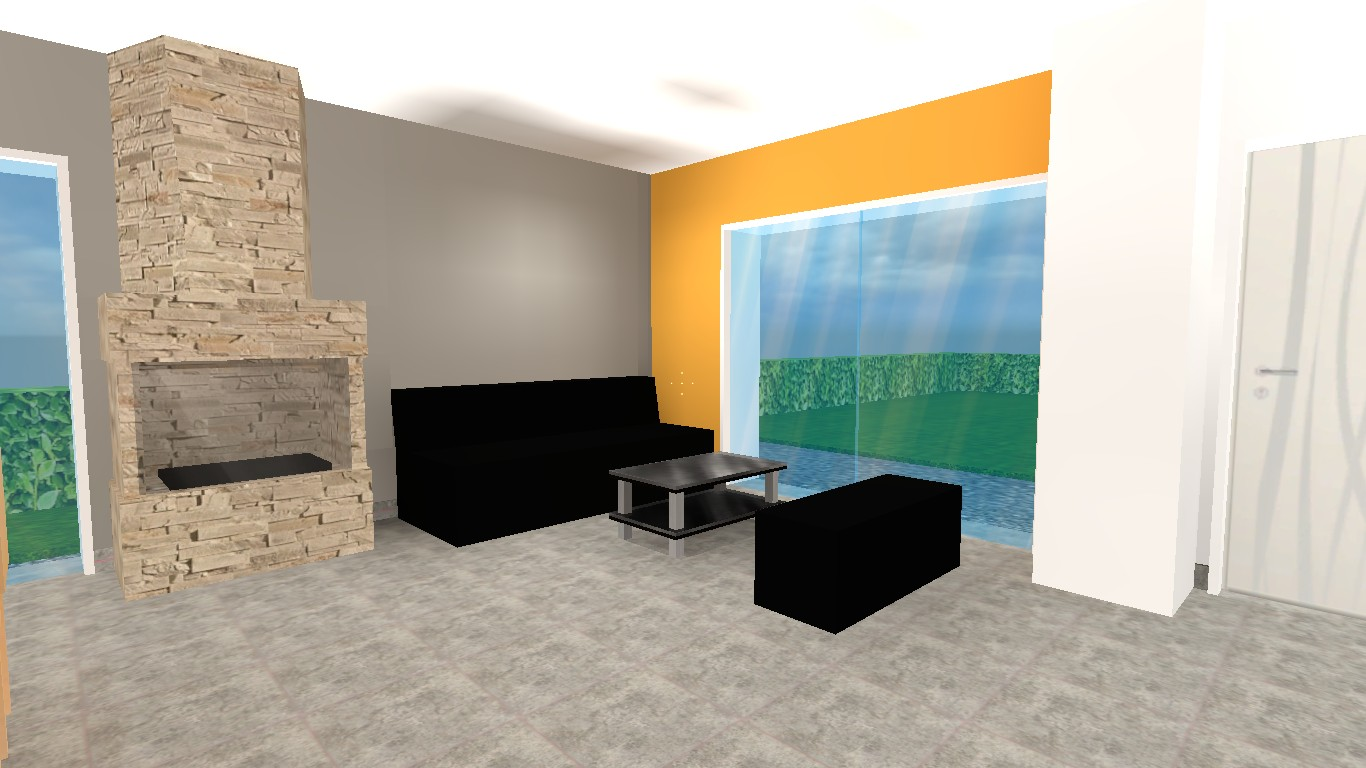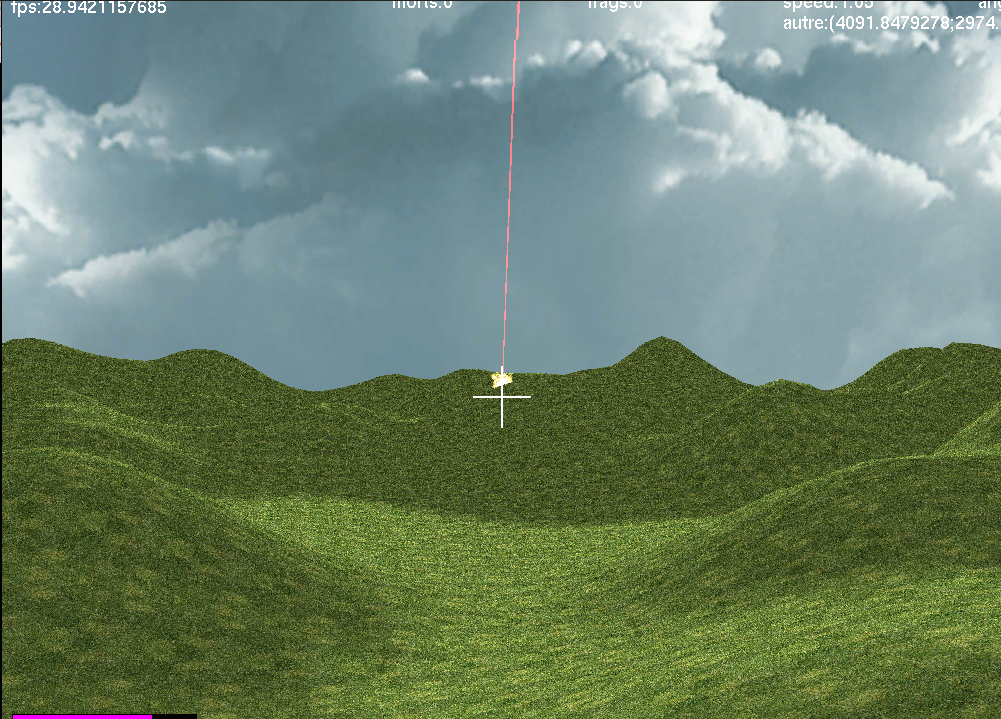# Vincent Gripon's Homepage

Research and Teaching Blog

## Graph Signal Processing

This webpage was coded by Chiraz Nafouki, during her internship at Télécom Bretagne.

Graph signal processing is a recent research domain that aim at generalizing classical signal processing. A graph is composed of a set of points, called nodes, such that some of them are connected through edges. If we label nodes from 1 to n, a graph is caracterized using an adjacency matrix. This matrix's cell (i,j) contains, if there is one, the connection weight connecting i and j, and 0 otherwise. A graph can be directed or not. An undirected graph has a symmetric adjacency matrix.
There are many application domains for graph signal processing. For instance the analysis of social networks, brain imaging, transport or power networks... Graphs are a generic model that can be applied everywhere.
Let us consider an example of a social network where nodes represent people and edges friendship relations between nodes:Example of a graph

## Graph signal

A graph signal is a vector that associates with each node of the graph a real value. For example the signal can be the temperatures measured by sensors at different locations (nodes).

### Representation in the spatial domain

There are many different ways to represent a signal in the spatial domain. Here we illustrate two of them: a 3D representation where signal values are depicted as bars with corresponding heights. The second example depicts signal values as color intensities. We use the latter in the following.2D representation

### Spectral domain representation

Another matrix associated with a graph is the normalized Laplician, defined as: L = I - D-1/2 A D-1/2, where I is the identity matrix, D is the degree matrix and A the adjacency matrix. This operator can measure the smoothness of a signal on a graph using its quadratic form. As a matter of fact, eigenvalues of this operator are equivalent to "discrete frequencies" of signal decomposition using discrete Fourrier Transform. In the foregoing we denote by λi the i-th eigenvalue of the normalized Laplician with λ0≤λ1≤...≤λn-1 , where n is the order of the graph.

## Graph signal diffusion

One can define a diffusion operator for signals supported by graphs. This matrix diffuses the signal so that asymptotically it almost surely converges to a nondegenerate value (neither infinity nor 0). Heat diffusion is one good example of this procedure.
The diffusion operator is W= D-1/2 A D-1/2 , where D is the degree matrix and A the adjacency matrix.
Given a graph and an initial signal on the graph, we propose to visualize the diffusion of the signal on the graph. For that, we multiply the signal iteratively by W.

### Initial signal (nonnegative values)

Noeud 0 : Noeud 1 :

Noeud 2 : Noeud 3 :

Noeud 4 : Noeud 5 :

Noeud 6 : Noeud 7 :

Noeud 8 :

#### Visualizing diffusion

Each time you click on "Diffuse!" the signal is multiplied by W. Obtained signal is represented both in space and frequency.## Home model using HL Source

Some views of a house construction project using Half-life source engine I realized.## FPS in OCaml

A screenshot of a first-person-shooter I coded using OCaml. The network is also functionnal :)## Personal Bépo layout

I use a modified bépo layout that I trained on my personal data.
You can find the console file here.
You can find the XKbmap here.
You can find the xmodmap file here.

## Research locations map

Display Research locations on a larger map

## Language

Vincent Gripon's Homepage